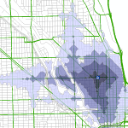# many to many shortest path, save as geometry

102
5
11-01-2022 10:15 AMby
Occasional Contributor III

Hi,

I have an OD matrix that defines flow between pairs of nodes. I would like to get the geometry of path(s) between these pairs of nodes. It is kind of paths between many to many nodes.  Is this possible with network analyst ?

Thank you.

1 Solution

Accepted SolutionsbyEsri Regular Contributor

Yes, I am trying many points as O and many points as D.

What is the "Yes" too? Are you doing an N by M OD matrix with all possible paths OR are you solving pairs of Origin to Destination routes? If you are doing a complete OD matrix then use the OD Cost Matrix solver. If you are doing pairs of O-D routes, then use the Route solver. Both the solvers are in the Network Analyst extension.

Jay Sandhu

Jay

5 RepliesbyEsri Regular Contributor

If you're doing an OD Cost Matrix analysis in ArcGIS, then it's not possible to retrieve the network path between each origin and destination.  The OD Cost Matrix solver uses the road network when calculating the travel times and distances, but it optimizes performance and memory usage by not storing those network paths.  It only stores the calculated costs, and it can optionally generate a straight line between each origin and destination.

If you want to get the geometry of the on-street routes between origins and destinations, you can use the Closest Facility solver instead.  The functionality is very similar to OD Cost Matrix, but it does have the option to store the geometry (and it's a little slower).  If you want to connect all origins to all destinations, you just need to set the number of facilities to find for the "closest" facility to a number >= your number of destinations.

Here's the Closest Facility tutorial: https://pro.arcgis.com/en/pro-app/latest/help/analysis/networks/closest-facility-tutorial.htmby
Occasional Contributor III

Thank you. So not much changed in many years of OD Cost Matrix. Thank you for confirming.byEsri Regular Contributor

Are you looking to generate N by M OD matrices? Or are you looking to generate paths between pairs of points? For pairs of points, the workflow is to use the Route solver with the ROUTENAME property.

Jay Sandhuby
Occasional Contributor III

Yes, I am trying many points as O and many points as D. They are the same set of points, but on a exteremely rare occasion can be partially different. I will look into route solver, is it also in the network extension?byEsri Regular Contributor

Yes, I am trying many points as O and many points as D.

What is the "Yes" too? Are you doing an N by M OD matrix with all possible paths OR are you solving pairs of Origin to Destination routes? If you are doing a complete OD matrix then use the OD Cost Matrix solver. If you are doing pairs of O-D routes, then use the Route solver. Both the solvers are in the Network Analyst extension.

Jay Sandhu

Jay